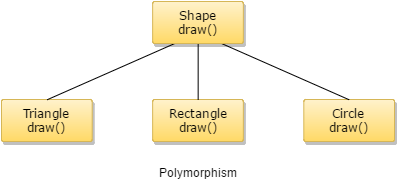## C++ Programming Tutorial Index

The term Polymorphism is derived from the Greek word poly + morphos, where poly means many and morphos means forms. This tutorial describes Polymorphism in C++.

## What is Polymorphism in C++

Polymorphism is another concept of object-oriented programming (OOPs). The attitude which lies beneath this concept is a "single interface having multiple implementations." This provides a single interface for controlling access to a general class of actions. Polymorphism can be gained in both ways:

• compile time and
• runtime

A common and simple example of Polymorphism is when you use `>>` and `<<` as operator overloading in C++ for `cin` and `cout` statements, respectively. This bitwise shift operator at that time acts as an inclusion operator, and its overloaded meaning is defined in the `iostream` header file.In the programming background, Polymorphism can be broadly divided into two parts. These are:
• Static Polymorphism
• Dynamic Polymorphism.

## Static Polymorphism

In static Polymorphism or early binding, there you will get two subcategories:

• Function overloading is the process of using the same name for two or more functions.
• Operator overloading is the process of using the same operator for two or more operands.

Example:

``````class funcOl {
public:
funcOl ();
funcOl (int i);
};``````

Example:

``````class calc {
public:
int operator+(calc g);
private:
int k;
};``````

It is to be noted that function overloading can be done and is possible only based on:

• The return type of that overloaded function.
• The type of parameters and their arrangement.
• Based on the number of parameters.

## Dynamic Polymorphism

Dynamic Polymorphism refers to the entity which changes its form depending on circumstances at runtime. This concept can be adopted as analogous to a chameleon changing its color at the sight of an approaching object.

## What Is Virtual Function?

A virtual function can be defined as the member function within a base class, which you expect to redefine in derived classes. To create a virtual function, you must precede your function's declaration within the base class with a `virtual` keyword.

#### Example of Using a Virtual Function

Example:

``````#include <iostream>
using namespace std;

class Game
{
int g;
public:
Game()
{
g = 1;
};

virtual void show()
{
cout <<g;
};
};

class Anim: public Game
{
int k;
public:

Anim()
{
k = 2;
};

virtual void show()
{
cout <<k;
};
};

int main() {
Game *g; Anim a; g = &a; g->show();
return 0;
}``````